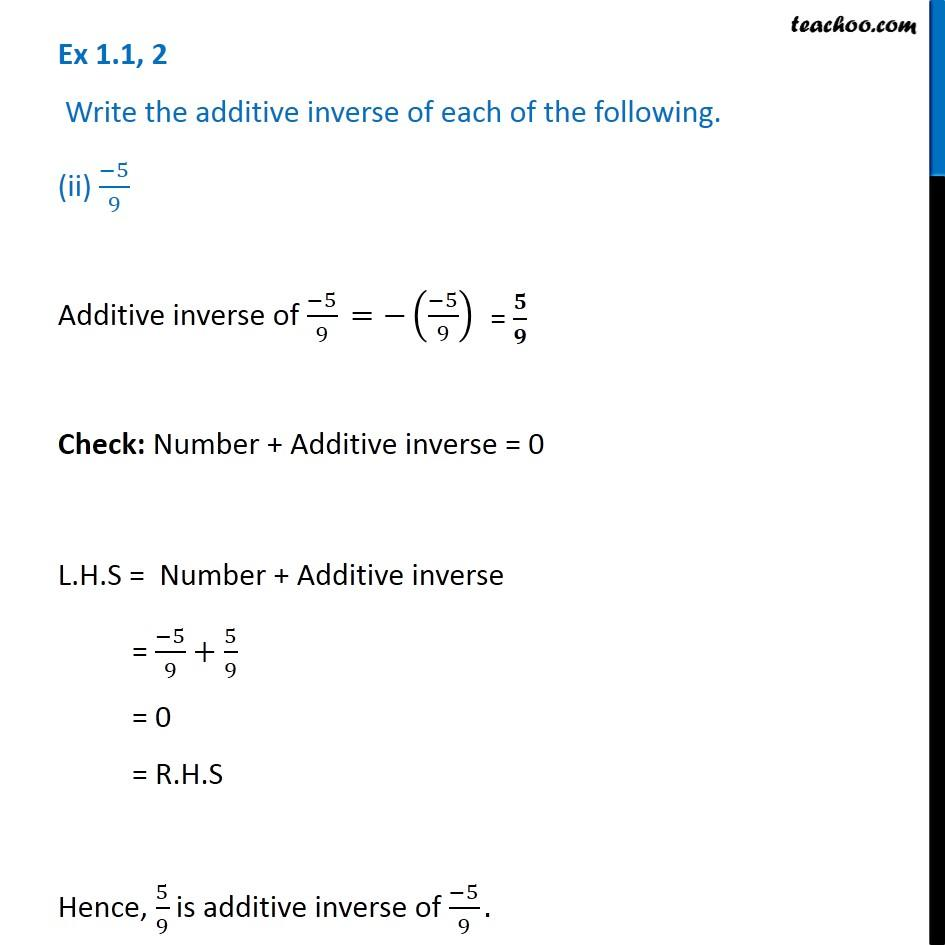Ex 1.1

Chapter 1 Class 8 Rational Numbers
Serial order wiseGet live Maths 1-on-1 Classs - Class 6 to 12

### Transcript

Ex 1.1, 2 Write the additive inverse of each of the following. (ii) ( 5)/9 Additive inverse of ( 5)/9 Check: Number + Additive inverse = 0 L.H.S = Number + Additive inverse = ( 5)/9+5/9 = 0 = R.H.S Hence, 5/9 is additive inverse of ( 5)/9.